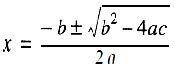Bsc. I.T. - Imperative Programming
Bsc. I.T.

### Imperative Programming Syllabus

 Unit Details I Introduction: Types of Programming languages, History, features and application. Simple program logic, program development cycle, pseudocode statements and flowchart symbols, sentinel value to end a program, programming and user environments, evolution of programming models., desirable program characteristics. Fundamentals: Structure of a program. Compilation and Execution of a Program, Character Set, identifiers and keywords, data types, constants, variables and arrays, declarations, expressions, statements, Variable definition, symbolic constants. II Operators and Expressions: Arithmetic operators, unary operators, relational and logical operators, assignment operators, assignment operators, the conditional operator, library functions. Data Input and output: Single character input and output, entering input data, scanf function, printf function, gets and puts functions, interactive programming. III Conditional Statements and Loops:Decision Making Within A Program, Conditions, Relational Operators, Logical Connectives, If Statement, If-Else Statement, Loops: While Loop, Do While, For Loop. Nested Loops, Infinite Loops, Switch Statement Functions: Overview, defining a function, accessing a function, passing arguments to a function, specifying argument data types, function prototypes, recursion, modular programming and functions, standard library of c functions, prototype of a function: foo1lal parameter list, return type, function call, block structure, passing arguments to a function: call by reference, call by value. IV Program structure: Storage classes, automatic variables, external variables, static variables, multifile programs, more library functions, Preprocessor: Features, #define and #include, Directives and Macros Arrays: Definition, processing, passing arrays to functions, multidimensional arrays, arrays and strings. V Pointers: Fundamentals, declarations, Pointers Address Operators, Pointer Type Declaration, Pointer Assignment, Pointer Initialization, Pointer Arithmetic, Functions and Pointers, Arrays And Pointers, Pointer Arrays, passing functions to other functions Structures and Unions: Structure Variables, Initialization, Structure Assignment, Nested Structure, Structures and Functions, Structures and Arrays: Arrays of Structures, Structures Containing Arrays, Unions, Structures and pointers.

### Imperative Programming Practicals

 Practical No Details 1 Basic Programs: a Write a program to display the message HELLO WORLD. b Write a program to declare some variables of type int, float and double. Assign some values to these variables and display these values. c Write a program to find the addition, subtraction, multiplication and division of two numbers. 2 Programs on variables: a Write a program to swap two numbers without using third variable. b Write a program to find the area of rectangle, square and circle. c Write a program to find the volume of a cube, sphere, and cylinder. 3 Conditional statements and loops(basic) a Write a program to enter a number from the user and display the month name. If number >13 then display invalid input using switch case. b Write a program to check whether the number is even or odd. c Write a program to check whether the number is positive, negative or zero. d Write a program to find the factorial of a number. e Write a program to check whether the entered number is prime or not. f Write a program to find the largest of three numbers. 4 Conditional statements and loops(advanced) a Write a program to find the sum of squares of digits of a number. b Write a program to reverse the digits of an integer. c Write a program to find the sum of numbers from 1 to 100. d Write a programs to print the Fibonacci series. e Write a program to find the reverse of a number. f Write a program to find whether a given number is palindrome or not. g Write a program that solve the quadratic equationh Write a program to check whether the entered number is Armstrong or not. i Write a program to count the digit in a number 5 Programs on patterns: a Programs on different patterns. 6 Functions. a Programs on Functions. 7 Recursive functions a Write a program to find the factorial of a number using recursive function. b Write a program to find the sum of natural number using recursive function. 8 Arrays a Write a program to find the largest value that is stored in the array. b Write a program using pointers to compute the sum of all elements stored in an array. c Write a program to arrange the ‘n’ numbers stored in the array in ascending and descending order. d Write a program that performs addition and subtraction of matrices. e Write a program that performs multiplication of matrices. 9 Pointers a Write a program to demonstrate the use of pointers. b Write a program to perform addition and subtraction of two pointer variables. 10 Structures and Unions a Programs on structures. b Programs on unions.

### Imperative Programming Reference Books

 Title Programming with C Authors Byron Gottfried Publisher Tata McGRAW-Hill Edition 2nd Year 1996 Download Here
 Title Programming Logic and Design Authors Joyce Farell Publisher Cengage Learning Edition 8th Year 2014 Download Here
 Title “C” Programming” Authors Brian W.Kernighan and Denis M.Ritchie. Publisher PHI Edition 2nd Year Download Here
 Title Let us C Authors Yashwant P.Kanetkar, Publisher BPB publication Edition Year Download Here